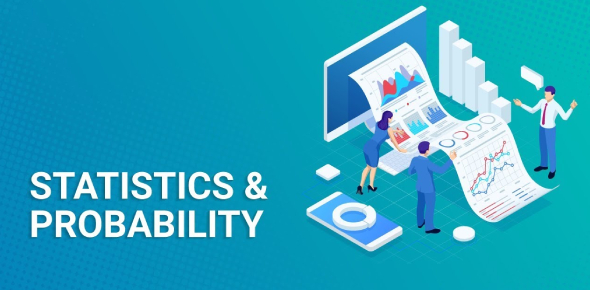# Statistics And Probability Quiz! Trivia

12 Questions | Total Attempts: 488Settings.

Related Topics
• 1.
The union of two events occurs when
• A.

Neither event A nor event B occur

• B.

Both event A and event B occur

• C.

Either event A or event B occur

• D.

Event A and event B are mutually exclusive

• E.

Event A and event B are independent

• 2.
The intersection of two events occurs when
• A.

Neither event A not event B occur

• B.

Both event A and event B occur

• C.

Either event A or event B occur

• D.

Event A and event B are mutually exclusive

• E.

Event A and event B are independent

• 3.
If events A and B are independent events
• A.

They occur simultaneously

• B.

Event B is not conditional on event A occurring

• C.

They are not influenced by one another

• D.

They cannot occur simultaneously

• E.

They are mutually exclusive

• 4.
If events A and B are mutually exclusive
• A.

They occur simultaneously

• B.

They cannot occur simultaneously

• C.

They are not influenced by one another

• D.

They are independent

• E.

Event B is conditional on event A occurring

• 5.
Bayes' Theorem
• A.

Gives the probability of two events occurring jointly

• B.

Gives the marginal probability of x.

• C.

Is a method for updating probabilities by using new information

• D.

Is a method for constructing Venn diagrams

• E.

Is a method for computing probabilities for mutually exclusive events

• 6.
The conditional probability of x given y is
• A.

The probability that x and y occur jointly

• B.

The probability that y occurs if x has already occurred

• C.

The probability that x occurs if y has already occurred.

• D.

The marginal probability of x minus the marginal probability of y.

• E.

The same as a joint probability.

• 7.
The probability of tossing three heads in a row is
• A.

1/2

• B.

1/4

• C.

1/8

• D.

1/6

• E.

1/16

• 8.
The probability of receiving one head and one tail in two flips of a coin is
• A.

The same as the probability of tossing two heads in a row

• B.

Is less than the probability of tossing two heads in a row

• C.

Is greater than the probability of tossing two heads in a row

• D.

Is 0

• E.

Is 1/2

• 9.
The multiplication rule in probability is only appropriate when the events
• A.

Are independent

• B.

Are mutually exclusive

• C.

Are dependent

• D.

Occur jointly

• E.

Are conditional upon one another

• 10.
If you roll two dice, the probability that the sum of the two rolls equals 7 is
• A.

1/36

• B.

2/36

• C.

1/6

• D.

3/36

• E.

1/4

• 11.
If you roll two dice, the probability that the sum of the two rolls equals 12 is
• A.

1/36

• B.

2/36

• C.

1/6

• D.

3/36

• E.

1/4

• 12.
Suppose the probability that you will be lected president of the sutdent body is .30, and the probabiliity that you will get into Harvard Law School is .05. If the two events are independent, the probability that you will not only become the student body president but also get into Harvard Law School is
• A.

.35

• B.

.25

• C.

.015

• D.

.50

• E.

.05